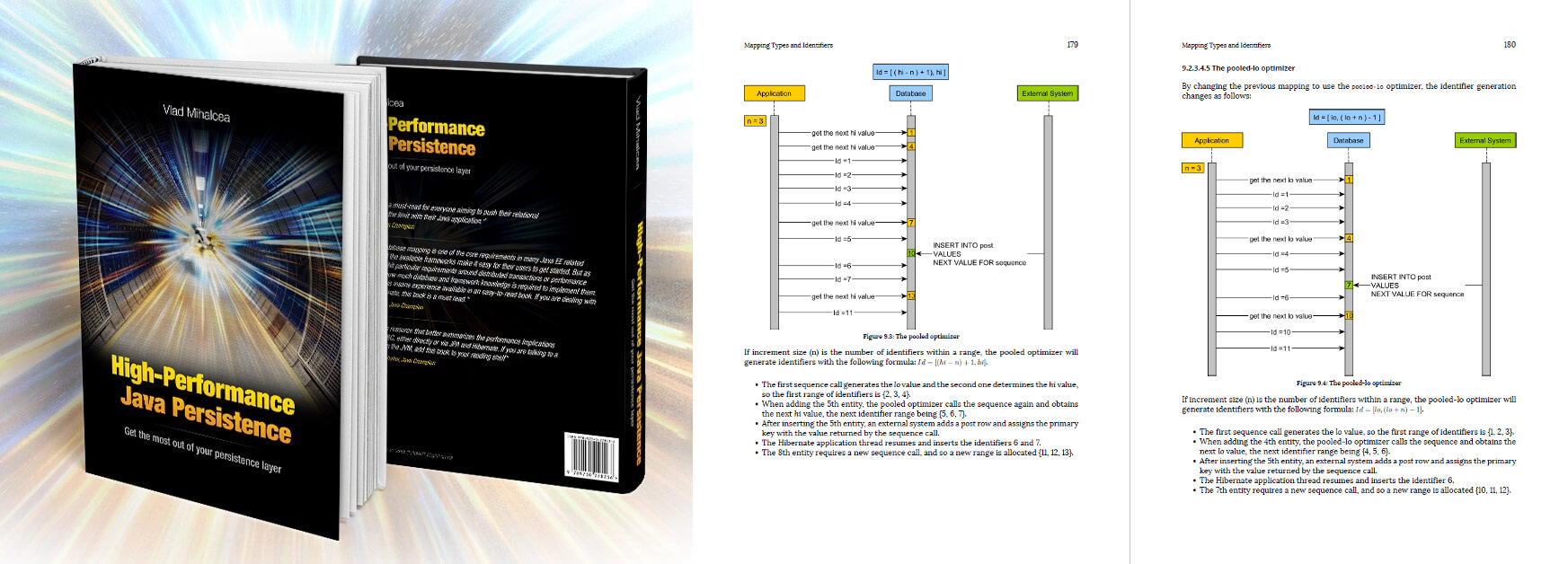# How to map calculated properties with JPA and Hibernate @Formula annotation

Imagine having a tool that can automatically detect if you are using JPA and Hibernate properly. Hypersistence Optimizer is that tool!

# Introduction

As I explained in this StackOverflow question, mapping calculated properties is very easy with JPA and Hibernate.

In this post, I’m going to demonstrate how you can derive some entity property based on one or multiple persistent entity attributes.

# Domain Model

Assuming we have the following `Attachment` entity mapping:The amount of money is stored in `cents` and there is an annual `interestRate` attribute which gives you how much you’ll gain for storing money in this saving account.

However, we need to calculate the following entity attributes based on the aforementioned `cents` and `interestRate`:

• `getDollars()` – gives you the deposit amount in dollars, not cents.
• `getInterestCents()` – gives you the interest in cents, accumulated since you started this saving account.
• `getInterestDollars()` – gives you the interest in dollars, accumulated since you started this saving account.

# Calculated properties using JPA

If you’re using JPA, you can implement these three methods as follows:

```@Transient
public double getDollars() {
return cents / 100D;
}

@Transient
public long getInterestCents() {
long months = createdOn.toLocalDateTime()
.until(LocalDateTime.now(), ChronoUnit.MONTHS);

double interestUnrounded = (
( interestRate / 100D ) * cents * months
)
/ 12;

return BigDecimal.valueOf(interestUnrounded)
.setScale(0, BigDecimal.ROUND_HALF_EVEN)
.longValue();
}

@Transient
public double getInterestDollars() {
return getInterestCents() / 100D;
}
```

Now, these properties are going to be calculated on every method call, and that might not be very efficient if you need to call a given method multiple times.

To overcome this issue, you can simply calculate these values upon loading the entity from the database (assuming the `cents` and the `interestRate` are not going to be modified since, otherwise, the saving `Account` will be deactivated).

Luckily, the JPA specification defines the `@PostLoad` entity listener which we can use for calculating these properties upon entity loading:

```@Transient
private double dollars;

@Transient
private long interestCents;

@Transient
private double interestDollars;

this.dollars = cents / 100D;

long months = createdOn.toLocalDateTime()
.until(LocalDateTime.now(), ChronoUnit.MONTHS);

double interestUnrounded = (
( interestRate / 100D ) * cents * months
)
/ 12;
this.interestCents = BigDecimal.valueOf(interestUnrounded)
.setScale(0, BigDecimal.ROUND_HALF_EVEN)
.longValue();

this.interestDollars = interestCents / 100D;
}

@Transient
public double getDollars() {
return dollars;
}

@Transient
public long getInterestCents() {
return interestCents;
}

@Transient
public double getInterestDollars() {
return interestDollars;
}
```

I marked all the non-persistent fields and properties as `@Transient` to make it clearer that these attributes are not supposed to be persisted by Hibernate.

# Calculated properties using Hibernate @Formula annotation

Hibernate offers the `@Formula` annotation, which can be used to calculate a given entity attribute using an SQL query expression:

```@Formula("cents::numeric / 100")
private double dollars;

@Formula(
"round(" +
"   (interestRate::numeric / 100) * " +
"   cents * " +
"   date_part('month', age(now(), createdOn)" +
") " +
"/ 12)")
private long interestCents;

@Formula(
"round(" +
"   (interestRate::numeric / 100) * " +
"   cents * " +
"   date_part('month', age(now(), createdOn)" +
") " +
"/ 12) " +
"/ 100::numeric")
private double interestDollars;

@Transient
public double getDollars() {
return dollars;
}

@Transient
public long getInterestCents() {
return interestCents;
}

@Transient
public double getInterestDollars() {
return interestDollars;
}
```

When fetching the `Account` entity, Hibernate is going to execute the following SQL query:

```Account account = entityManager.find(Account.class, 1L);
```
```SELECT a.id AS id1_0_0_,
a.cents AS cents2_0_0_,
a.createdOn AS createdO3_0_0_,
a.iban AS iban4_0_0_,
a.interestRate AS interest5_0_0_,
a."owner_id" AS owner_id6_0_0_,
a.cents::numeric / 100 AS formula0_0_,
round((a.interestRate::numeric / 100) * a.cents * date_part('month', age(now(), a.createdOn)) / 12) AS formula1_0_,
round((a.interestRate::numeric / 100) * a.cents * date_part('month', age(now(), a.createdOn)) / 12) / 100::numeric AS formula2_0_
FROM account a
WHERE a.id = 1
```

That’s it!

# Testing time

Assuming we have the following `Account` entity:

```doInJPA(entityManager -> {
User user = new User();
user.setId(1L);
user.setFirstName("John");
user.setFirstName("Doe");

entityManager.persist(user);

Account account = new Account(
1L,
user,
"ABC123",
12345L,
6.7,
Timestamp.valueOf(
LocalDateTime.now().minusMonths(3)
)
);
entityManager.persist(account);
});
```

We can validate the calculated properties as follows:

```doInJPA(entityManager -> {
Account account = entityManager.find(Account.class, 1L);

assertEquals(123.45D, account.getDollars(), 0.001);
assertEquals(207L, account.getInterestCents());
assertEquals(2.07D, account.getInterestDollars(), 0.001);
});
```

Code available on GitHub.

If you enjoyed this article, I bet you are going to love my Book and Video Courses as well.

# Conclusion

Mapping calculated entity properties is fairly easy with both JPA and Hibernate, and you should definitely take advantage of this feature.

FREE EBOOK### 2 Comments on “How to map calculated properties with JPA and Hibernate @Formula annotation”

1.sanjeev kumar jha

Hi

•vladmihalcea
The `::` cast operator is PostgreSQL-specific while you are running on MySQL. Use the `CAST` function instead.